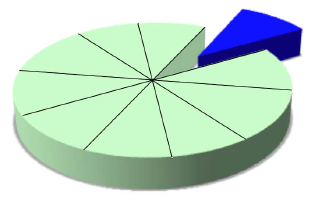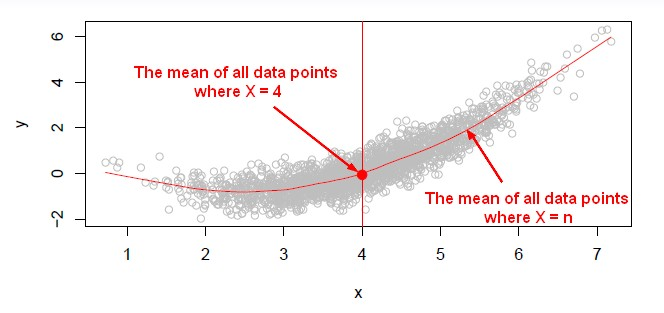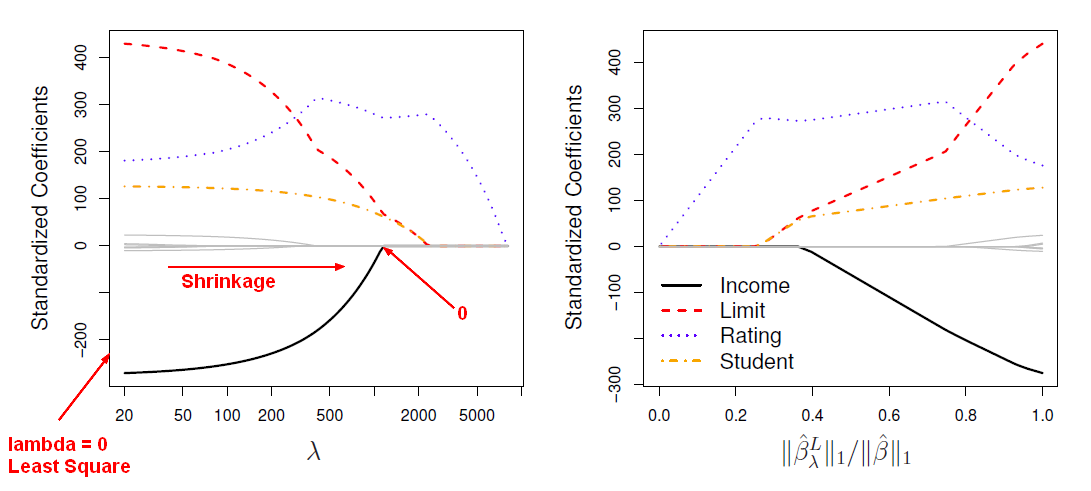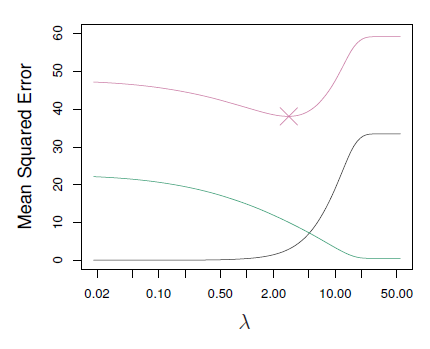# Data Mining - (Attribute|Feature) (Selection|Importance)

Feature selection is the second class of dimension reduction methods. They are used to reduce the number of predictors used by a model by selecting the best d predictors among the original p predictors.

This allows for smaller, faster scoring, and more meaningful Generalized Linear Models (GLM).

Feature selection techniques are often used in domains where there are many features and comparatively few samples (or data points).

Feature selection is also useful as part of the data analysis process, as it shows which features are important for prediction, and how these features are related.

What are the important variables to include in the model.

When we have a small number of features, the model becomes more interpretable.

Feature selection is a way of choosing among features to find the ones that are most informative.

We'd like to fit a model that has all the good (signal) variables and leaves out the noise variables.

Feature selections procedure generate models and uses models_selection methods to try to find among the p predictors the ones that are the most related to the response.

## Assumption

The central assumption when using a feature selection technique is that the data contains many redundant or irrelevant features.

## Procedure

We have access to p predictors but we want to actually have a simpler model that involves only a subset of those p predictors. This model selection is made in two steps:

• model generation and selection for each k parameters
• model selection among all best model for each k parameters. We choose then between them based on some criterion that balances training error with model size.

### Models Generation

#### Subset selection

All the below methods take a subset of the predictors and use least squares to fit the model.

Subset selection flavours two methods in order to generate the models for k predictors:

#### Shrinkage

The shrinkage methods take all of the predictors, but use a shrinkage approach to fit the model instead of least squares.

The shrinkage approach fit the coefficients by minimizing the RSS (as for least squares) but with a penalty term. The regression coefficients will then shrink towards, typically, 0.

#### Dimension reduction

This methods use least squares not on the original predictors but on new predictors, which are linear combinations of the original projectors.

## Documentation / Reference

Recommended Pages(Statistics|Data Mining) - (K-Fold) Cross-validation (rotation estimation)

Cross-validation, sometimes called rotation estimation is a resampling validation technique for assessing how the results of a statistical analysis will generalize to an independent new data set. This...Data Mining - (Dimension|Feature) (Reduction)

In machine learning and statistics, dimensionality reduction is the process of reducing the number of random variables (features) under consideration and can be divided into: feature selection (returns...Data Mining - Dimensionality (number of variable, parameter) (P)

Not to confound with d: the model size. You may have 1000 attributes (p=1000) in your sample but after feature selection for instance, you model may use only a handful (d=5) In physics and mathematics,...Machine Learning - K-Nearest Neighbors (KNN) algorithm - Instance based learning

“Nearest‐neighbor” learning is also known as “Instance‐based” learning. K-Nearest Neighbors, or KNN, is a family of simple: classification and regression algorithms based on Similarity...R - Feature Selection - Indirect Model Selection

In a feature selection process, once you have generated all possible models, you have to select the best one. This article talks the indirect methods. We will select the models using CP but as...R - Feature Selection - Model selection with Direct validation (Validation Set or Cross validation)

Feature selection through model generation and selection through a direct approach with : validation set and cross validation in R We are picking a subset of the observations and put them...R - Feature selection - Model Generation (Best Subset and Stepwise)

This article talks the first step of feature selection in R that is the models generation. Once the models are generated, you can select the best model with one of this approach: Best subset regressiosigreedy...Statistical Learning - Lasso

Lasso is a shrinkage method. Ridge regression doesn't actually select variables by settings the parameters to zero. Lasso is a more recent technique for shrinking coefficients in regression that overcomes...Statistics - (Shrinkage|Regularization) of Regression Coefficients

Shrinkage methods are more modern techniques in which we don't actually select variables explicitly but rather we fit a model containingall p predictors using a technique that constrains or regularizes...Statistics - Bayesian Information Criterion (BIC)

BIC is like AIC and Mallow's Cp, but it comes from a Bayesian argument. The formulas are very similar. The formula calculate the residual sum of squares and then add anadjustment term which is...